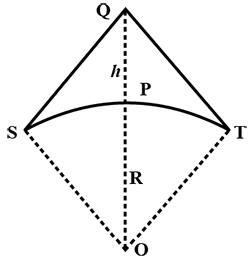Courses

# Test: Signals And Transmission Systems

## 10 Questions MCQ Test Physics Class 12 | Test: Signals And Transmission Systems

Description
This mock test of Test: Signals And Transmission Systems for JEE helps you for every JEE entrance exam. This contains 10 Multiple Choice Questions for JEE Test: Signals And Transmission Systems (mcq) to study with solutions a complete question bank. The solved questions answers in this Test: Signals And Transmission Systems quiz give you a good mix of easy questions and tough questions. JEE students definitely take this Test: Signals And Transmission Systems exercise for a better result in the exam. You can find other Test: Signals And Transmission Systems extra questions, long questions & short questions for JEE on EduRev as well by searching above.
QUESTION: 1

### The characteristic impedance of a coaxial wire line is of order

Solution:

For a coaxial cable with air dielectric and a shield of a given inner diameter, the attenuation is minimized by choosing the diameter of the inner conductor to give a characteristic impedance of 76.7 Ω. When more common dielectrics are considered, the best-loss impedance drops down to a value between 52–64 Ω.

QUESTION: 2

### In which manner does current or voltage vary in an analog signal with time?

Solution:

An analog signal is any continuous signal for which the time-varying feature (variable) of the signal is a representation of some other time-varying quantity, i.e., analogous to another time-varying signal. For example, in an analog audio signal, the instantaneous voltage of the signal varies continuously with the pressure of the sound waves. It differs from a digital signal, in which the continuous quantity is a representation of a sequence of discrete values which can only take on one of a finite number of values.

QUESTION: 3

### If an antenna radiates electromagnetic waves from a height h, then the range d upto which the signal can be detected is given by (R is the radius of the earth)​

Solution:

Let O be the centre and R is the radius of the earth. PQ is a T.V. antenna of height h. Two tangents QT and QS are drawn from the top Q of the tower. T.V. signal can reach to the point S and T only not at other area:
∠QTO=90[TO⊥QT]
In ΔQTO,
OQ2=OT2+TQ2
OQ=R+h,  QT=d,  OT=R,
(R+h)2=R2+d2
R2+h2+2Rh=R2+d2
d2=h2+2Rh  h⋘R neglect
d2=2Rh
d= √2RhQUESTION: 4

Super high frequencies of __________ are used in Radar and Satellite Communication.​

Solution:

Super high frequency (SHF) is the ITU designation for radio frequencies (RF) in the range between 3 and 30 gigahertz (GHz). This band of frequencies is also known as the centimetre band or centimetre wave as the wavelengths range from one to ten centimetres.

QUESTION: 5

The bandwidth sufficient for commercial telephonic communication:​

Solution:

For speech signals (as in telephone) frequency range 300 Hz- 3100 Hz is adequate i.e., a speech signal requires a bandwidth of 2800 Hz.

QUESTION: 6

Optical communication using fibres is performed in the frequency range of​

Solution:

Optical communication using fibers is performed in the frequency range of 1 THz to 1000 THz (microwaves to ultraviolet). An optical fiber can offer a transmission bandwidth in excess of 100 GHz.

QUESTION: 7

Modes of communication system are classified into :

Solution:
QUESTION: 8

If the area of a TV telecast is to be doubled, then height of transmitting antenna will have to be

Solution:

Distance of receiving antenna a from transmitting antenna is d = √(2Rh) i.e. d ∝ √h area covered ∝ d2 that is area covered ∝ h. Hence if the area is double, the height of the antenna will also be doubled.

QUESTION: 9

With the increase in frequencies more than 2 MHz, Ground wave propagation

Solution:

The ground wave is the preferred propagation type for long distance communication using frequencies below 3 MHz (the earth behaves as a conductor for all frequencies below 5 MHz). The ground wave is also used for short distance communications using frequencies between 3 and 30 MHz.

QUESTION: 10

What should be the approximate height of antenna if the TV telecast is to cover a radius of 100 km?

Solution:

Here , d=100km=100×103m,
R=6.4×106m
d=√2Rh
or h=d2/2R=(100×103)2/2×6.4×106=781.25m